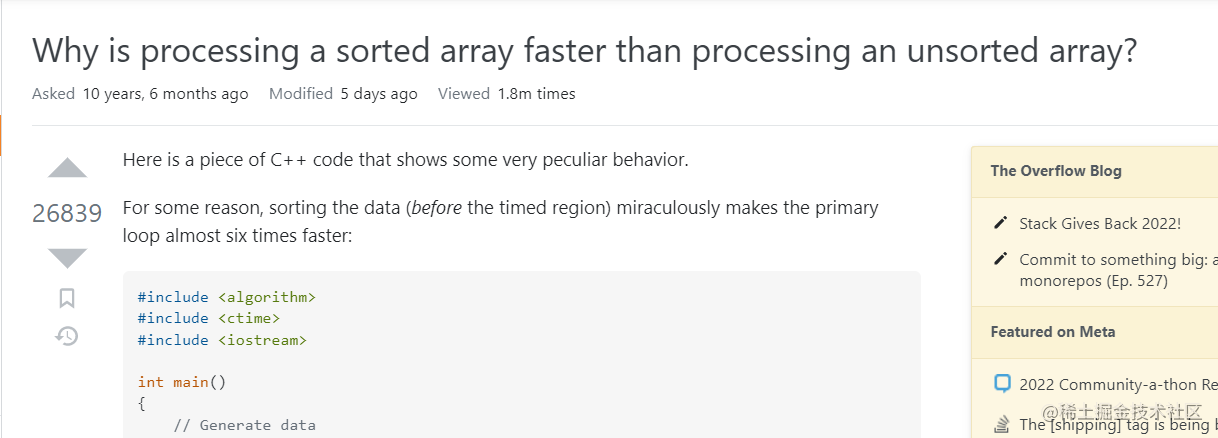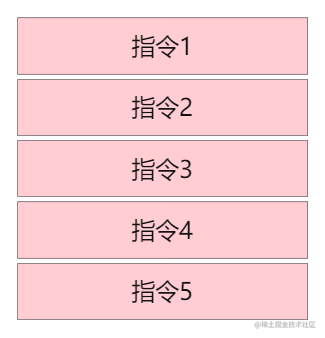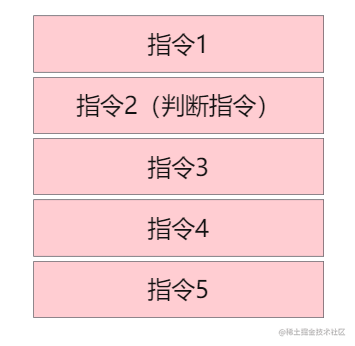# 为什么处理一个排好序的数组比未排序的数组要快？1. 生成随机数放入数组
2. 对数组进行排序
3. 对数组中的数字进行累加
``````public class Main {
public static void main(String[] args) {
// 生成随机数
int arraySize = 32768;
int data[] = new int[arraySize];

Random rnd = new Random(0);
for (int c = 0; c < arraySize; ++c)
data[c] = rnd.nextInt() % 256;

// 排序
Arrays.sort(data);

// 累加，测试执行时间
long start = System.nanoTime();
long sum = 0;
for (int i = 0; i < 100000; ++i) {
for (int c = 0; c < arraySize; ++c) {
if (data[c] >= 128)
sum += data[c];
}
}

System.out.println((System.nanoTime() - start) / 1000000000.0);
System.out.println("sum = " + sum);
}
}``````for (int c = 0; c < arraySize; ++c) {
if (data[c] >= 128)
sum += data[c];
}

if语句就相当于是一个判断指令，根据判断结果就会有两个分支：

1. 要么进行累加
2. 要么取数组中的下一个数字。

1. 比如预测当前判断的数字是大于128的，那么就可以在判断结果没有出来之前提前进行累加
2. 或者预测当前判断的数字是小于128的，那么就可以在判断结果没有出来之前提前取下一个数字